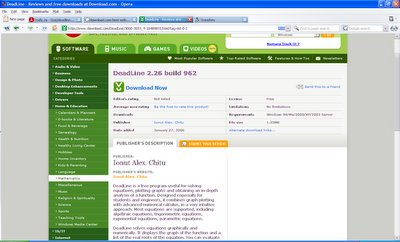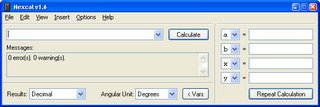## 27 January, 2006## 09 December, 2005

### Nexcal, numerical expression calculator

Nexcal is meant to be an advanced yet fast and easy to use calculator, suitable for a variety of computations. What separates Nexcal from many simpler calculators, is among other things that Nexcal can compute complete mathematical expressions, rather than simply performing one operation at a time.

Some of the features:

* Includes over 30 different operators such as sin, cos, tan, mod, ln etc.

* Can handle variables containing either single values or complete expressions.

* Includes functions for equation solving, unit conversion, prime number searching, calculating greatest common divisor / least common multiple and more.

* Support for decimal, binary and hexadecimal numbers, that can be mixed freely within an expression.## 07 November, 2005

### Fahrenheit to Celsius conversion formula

The formula below shows how temperatures on the Fahrenheit scale relates to the Celsius scale.

Tc = (5/9)*(Tf-32)
Tc = temperature in degrees Celsius
Tf = temperature in degrees Fahrenheit

For example, suppose you have a Fahrenheit temperature of 98.6 degrees and you wanted to convert it into degrees Celsius. Using the above formula, you would first subtract 32 from the Fahrenheit temperature and get 66.6. Then you multiply 66.6 by five-ninths and get 37 degrees Celsius.

The formula to convert a Celsius temperature into degrees Fahrenheit is:

Tf = (9/5)*Tc+32
Tc = temperature in degrees Celsius
Tf = temperature in degrees Fahrenheit

For example, suppose you have a Celsius temperature of 100 degrees and you want to convert it into degrees Fahrenheit. Using the above formula, you first multiply the Celsius temperature reading by nine-fifths and get 180. Then you add 32 to 180 and get 212 degrees Fahrenheit.

How can you convert Fahrenheit to Celsius using DeadLine Calculator?

Define the function:
f2c:(5/9)*(x-32)

Then make the conversion:
f2c(98.6)

The result will be 37 degrees Celsius.

To convert Celsius to Fahrenheit, define another function:

c2f:(9/5)*x+32

c2f(100)
will display 212 degrees Fahrenheit.

## 27 October, 2005The newest version of DeadLine Equation Solver brings you a new way of solving equations: define parameters and change their values to instantly graph and solve similar equations.

See an illustration for solving parametric equations.

## 17 September, 2005

It's so easy to translate DeadLine in your own language that it won't take you more than 10 minutes. Just edit a text file in your favourite text editor (I recommend Notepad2).

Una gran ayuda para tus deberes de matemáticas.

## 11 September, 2005

If something goes wrong with DeadLine, if you delete a file or a shortcut, you can always repair the installation very easily.

Open Control Panel / Add or Remove Programs, select DeadLine Equation Solver from the list and click on Change button. You'll notice a trim-down version of the setup that reinstalls the software.

## 30 August, 2005

### As good as it gets

It's so easy to close your eyes and pretend it didn't happen. Find a miracle and ignore it, just because you don't want to get involved.

There are so many miraculous things around us and we don't see them. Math is one of those miracles.